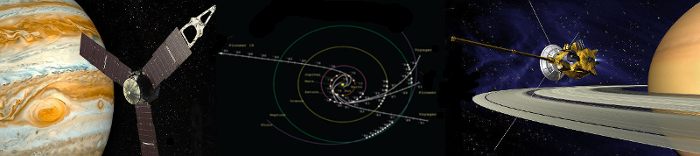Record Solutions on Space Mission BenchmarksSince 2005 the Advanced Concept Team (ACT) of the the European Space Agency (ESA) makes available a set of challenging space mission benchmarks, known as the GTOP database. MIDACO holds currently the record solutions on the GTOC1, Cassini2 and Messenger (Full Version) instances. The Messenger problem is known to be the hardest benchmark in this set. Note that the total time between the first and last solution submission on this benchmark took over 4.5 Years. MIDACO currently holds the first, second and third record solution on this benchmark, whereas the first and second record solution is very close to the original Messenger Trajectory launched by NASA in 2004.

 Record Nr. 1 * *   (Apr 2014) Record Nr. 2   (Feb 2014) Record Nr. 3    (Nov 2013) 1.972  km/sec 1.983  km/sec 2.104  km/sec x[   0] = 2037.735811; x[   1] = 4.036904766; x[   2] = 0.555556999; x[   3] = 0.636428972; x[   4] = 451.4843821; x[   5] = 224.6942632; x[   6] = 221.8566317; x[   7] = 265.9333414; x[   8] = 357.9769956; x[   9] = 534.1065761; x[ 10] = 0.532515156; x[ 11] = 0.706442511; x[ 12] = 0.718411658; x[ 13] = 0.759622141; x[ 14] = 0.828618506; x[ 15] = 0.902851485; x[ 16] = 1.424762657; x[ 17] = 1.100000006; x[ 18] = 1.050585900; x[ 19] = 1.050000000; x[ 20] = 1.050000000; x[ 21] = 2.820002913; x[ 22] = 1.513622824; x[ 23] = 2.593053687; x[ 24] = 1.754040819; x[ 25] = 1.568520102; x[   0] = 2037.777752;x[   1] = 4.036037772;x[   2] = 0.555461305;x[   3] = 0.636411860;x[   4] = 451.4564248;x[   5] = 224.6943091;x[   6] = 221.8810790;x[   7] = 265.2452367;x[   8] = 358.3268877;x[   9] = 534.1843070;x[ 10] = 0.599458381;x[ 11] = 0.739157714;x[ 12] = 0.719150322;x[ 13] = 0.759748648;x[ 14] = 0.828459261;x[ 15] = 0.902545986;x[ 16] = 1.424681465;x[ 17] = 1.100000319;x[ 18] = 1.051121973;x[ 19] = 1.150030195;x[ 20] = 1.050000606;x[ 21] = 2.820044490;x[ 22] = 1.514855471;x[ 23] = 2.589799685;x[ 24] = 1.756472273;x[ 25] = 1.561537932; x[   0] = 2060.627272;x[   1] = 4.042601735;x[   2] = 0.440387114;x[   3] = 0.653458177;x[   4] = 428.9035253;x[   5] = 224.6872358;x[   6] = 221.3854274;x[   7] = 266.1243673;x[   8] = 358.0485999;x[   9] = 444.4294274;x[ 10] = 0.581561467;x[ 11] = 0.821640755;x[ 12] = 0.698772357;x[ 13] = 0.720609016;x[ 14] = 0.829340143;x[ 15] = 0.875166415;x[ 16] = 1.576183861;x[ 17] = 1.100003220;x[ 18] = 1.052869945;x[ 19] = 1.050000430;x[ 20] = 1.477180737;x[ 21] = 2.786201469;x[ 22] = 1.603649010;x[ 23] = 2.622074959;x[ 24] = 1.571933956;x[ 25] = 1.606318012; * Update 15/09/2014:   The MIDACO extension MXHPC obtained a new record of 1.959 * Update 6/09/2017:   Mingcheng Zuo obtained a new record of 1.958 by refinement

A contribution especially dedicated to the Messenger (Full Mission) benchmark:

Schlueter M, Wahib M., Munetomo M.

Numerical optimization of ESA’s Messenger space mission benchmark

Proc. Evostar Conference, Amsterdam 19-21 April, accepted (2017)

A comprehensive study of MIDACO 4.0 on the ESA-GTOP benchmark suite can be found in:

Schlueter, M.:

MIDACO Software Performance on Interplanetary Trajectory Benchmarks

Advances in Space Research (Elsevier), Volume 54, Issue 4, Pages 744–754 (2014)

Accompanying source codes and log-files:

 Makefile (gcc & g++) Source Code (C/C++) Screenshots (as .txt) Makefile main.cpp cassini1.txt gtoc1.txt messenger.txt messengerfull.txt cassini2.txt rosetta.txt sagas.txt

 Makefile (gcc & g++) Source Code (C/C++) Screenshots (as .txt) Makefile main.cpp cassini1.txt messenger.txt messengerfull.txt cassini2.txt rosetta.txt sagas.txt

 Cassini1 cassini1.txt GTOC1 gtoc1.txt Messenger (reduced) messenger.txt Messenger (full) messenger_full.txt Cassini2 cassini2.txt Sagas sagas.txt Rosetta rosetta.txt